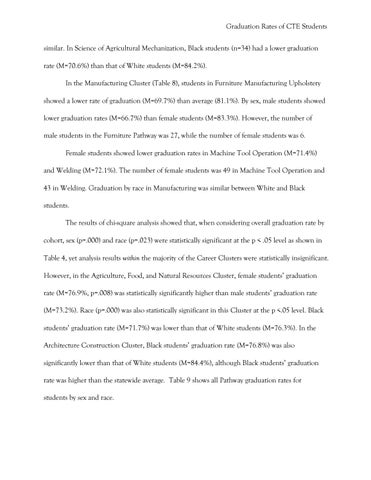Graduation Rates of CTE Students similar. In Science of Agricultural Mechanization, Black students (n=34) had a lower graduation rate (M=70.6%) than that of White students (M=84.2%). In the Manufacturing Cluster (Table 8), students in Furniture Manufacturing Upholstery showed a lower rate of graduation (M=69.7%) than average (81.1%). By sex, male students showed lower graduation rates (M=66.7%) than female students (M=83.3%). However, the number of male students in the Furniture Pathway was 27, while the number of female students was 6. Female students showed lower graduation rates in Machine Tool Operation (M=71.4%) and Welding (M=72.1%). The number of female students was 49 in Machine Tool Operation and 43 in Welding. Graduation by race in Manufacturing was similar between White and Black students. The results of chi-square analysis showed that, when considering overall graduation rate by cohort, sex (p=.000) and race (p=.023) were statistically significant at the p < .05 level as shown in Table 4, yet analysis results within the majority of the Career Clusters were statistically insignificant. However, in the Agriculture, Food, and Natural Resources Cluster, female students’ graduation rate (M=76.9%, p=.008) was statistically significantly higher than male students’ graduation rate (M=73.2%). Race (p=.000) was also statistically significant in this Cluster at the p <.05 level. Black students’ graduation rate (M=71.7%) was lower than that of White students (M=76.3%). In the Architecture Construction Cluster, Black students’ graduation rate (M=76.8%) was also significantly lower than that of White students (M=84.4%), although Black students’ graduation rate was higher than the statewide average. Table 9 shows all Pathway graduation rates for students by sex and race.

Graduation Rates and Contributing Factors in CTE Students

Published in Proceedings of 2013 CREATE Conference Authors: Young Bin Lim Sean Owen Alexis Nordin

Graduation Rates and Contributing Factors in CTE Students

Published in Proceedings of 2013 CREATE Conference Authors: Young Bin Lim Sean Owen Alexis Nordin